Next: Ampère's law Up: Time-independent Maxwell equations Previous: Ampère's experiments

## The Lorentz force

The flow of an electric current down a conducting wire is ultimately due to the motion of electrically charged particles (in most cases, electrons) through the conducting medium. It seems reasonable, therefore, that the force exerted on the wire when it is placed in a magnetic field is really the resultant of the forces exerted on these moving charges. Let us suppose that this is the case.

Letbe the (uniform) cross-sectional area of the wire, and let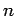be the number density of mobile charges in the conductor. Suppose that the mobile charges each have charge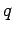and velocity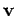. We must assume that the conductor also contains stationary charges, of chargeand number density(say), so that the net charge density in the wire is zero. In most conductors, the mobile charges are electrons and the stationary charges are atomic nuclei. The magnitude of the electric current flowing through the wire is simply the number of coulombs per second which flow past a given point. In one second, a mobile charge moves a distance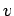, so all of the charges contained in a cylinder of cross-sectional areaand lengthflow past a given point. Thus, the magnitude of the current is. The direction of the current is the same as the direction of motion of the charges, so the vector current is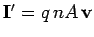. According to Eq. (229), the force per unit length acting on the wire is(232)

However, a unit length of the wire contains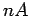moving charges. So, assuming that each charge is subject to an equal force from the magnetic field (we have no reason to suppose otherwise), the force acting on an individual charge is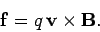(233)

We can combine this with Eq. (169) to give the force acting on a chargemoving with velocityin an electric field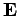and a magnetic field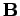: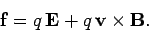(234)

This is called the Lorentz force law, after the Dutch physicist Hendrik Antoon Lorentz who first formulated it. The electric force on a charged particle is parallel to the local electric field. The magnetic force, however, is perpendicular to both the local magnetic field and the particle's direction of motion. No magnetic force is exerted on a stationary charged particle.

The equation of motion of a free particle of chargeand massmoving in electric and magnetic fields is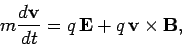(235)

according to the Lorentz force law. This equation of motion was first verified in a famous experiment carried out by the Cambridge physicist J.J. Thompson in 1897. Thompson was investigating cathode rays, a then mysterious form of radiation emitted by a heated metal element held at a large negative voltage (i.e., a cathode) with respect to another metal element (i.e., an anode) in an evacuated tube. German physicists held that cathode rays were a form of electromagnetic radiation, whilst British and French physicists suspected that they were, in reality, a stream of charged particles. Thompson was able to demonstrate that the latter view was correct. In Thompson's experiment, the cathode rays passed though a region of crossed'' electric and magnetic fields (still in vacuum). The fields were perpendicular to the original trajectory of the rays, and were also mutually perpendicular.

Let us analyze Thompson's experiment. Suppose that the rays are originally traveling in the-direction, and are subject to a uniform electric fieldin the-direction and a uniform magnetic fieldin the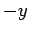-direction. Let us assume, as Thompson did, that cathode rays are a stream of particles of massand charge. The equation of motion of the particles in the-direction is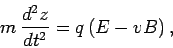(236)

whereis the velocity of the particles in the-direction. Thompson started off his experiment by only turning on the electric field in his apparatus, and measuring the deflectionof the ray in the-direction after it had traveled a distance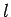through the electric field. It is clear from the equation of motion that(237)

where the time of flight''is replaced by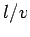. This formula is only valid if, which is assumed to be the case. Next, Thompson turned on the magnetic field in his apparatus, and adjusted it so that the cathode ray was no longer deflected. The lack of deflection implies that the net force on the particles in the-direction was zero. In other words, the electric and magnetic forces balanced exactly. It follows from Eq. (236) that with a properly adjusted magnetic field strength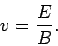(238)

Thus, Eqs. (237) and (238) and can be combined and rearranged to give the charge to mass ratio of the particles in terms of measured quantities: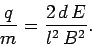(239)

Using this method, Thompson inferred that cathode rays were made up of negatively charged particles (the sign of the charge is obvious from the direction of the deflection in the electric field) with a charge to mass ratio of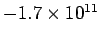C/kg. A decade later, in 1908, the American Robert Millikan performed his famous oil drop'' experiment, and discovered that mobile electric charges are quantized in units of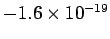C. Assuming that mobile electric charges and the particles which make up cathode rays are one and the same thing, Thompson's and Millikan's experiments imply that the mass of these particles iskg. Of course, this is the mass of an electron (the modern value iskg), andC is the charge of an electron. Thus, cathode rays are, in fact, streams of electrons which are emitted from a heated cathode, and then accelerated because of the large voltage difference between the cathode and anode.

Consider, now, a particle of massand chargemoving in a uniform magnetic field,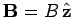. According, to Eq. (235), the particle's equation of motion can be written: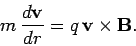(240)

This reduces to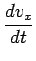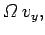(241)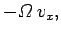(242)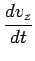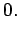(243)

Here,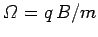is called the cyclotron frequency. The above equations can be solved to give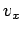(244)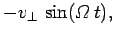(245)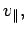(246)

and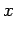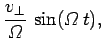(247)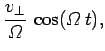(248)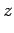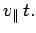(249)

According to these equations, the particle trajectory is a spiral whose axis is parallel to the magnetic field. The radius of the spiral is, whereis the particle's constant speed in the plane perpendicular to the magnetic field. The particle drifts parallel to the magnetic field at a constant velocity,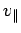. Finally, the particle gyrates in the plane perpendicular to the magnetic field at the cyclotron frequency.

Finally, if a particle is subject to a force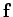and moves a distancein a time interval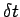, then the work done on the particle by the force is(250)

The power input to the particle from the force field is(251)

whereis the particle's velocity. It follows from the Lorentz force law, Eq. (234), that the power input to a particle moving in electric and magnetic fields is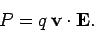(252)

Note that a charged particle can gain (or lose) energy from an electric field, but not from a magnetic field. This is because the magnetic force is always perpendicular to the particle's direction of motion, and, therefore, does no work on the particle [see Eq. (250)]. Thus, in particle accelerators, magnetic fields are often used to guide particle motion (e.g., in a circle) but the actual acceleration is performed by electric fields.Next: Ampère's law Up: Time-independent Maxwell equations Previous: Ampère's experiments
Richard Fitzpatrick 2006-02-02